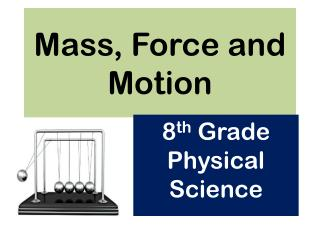# Mass, Force and Motion - PowerPoint PPT PresentationDownload PresentationMass, Force and Motion

Mass, Force and MotionDownload Presentation## Mass, Force and Motion

- - - - - - - - - - - - - - - - - - - - - - - - - - - E N D - - - - - - - - - - - - - - - - - - - - - - - - - - -
##### Presentation Transcript

1. Mass, Force and Motion 8th Grade Physical Science

2. S8P3. Students will investigate the relationship between force, mass, and the motion of objects. Demonstrate the effect of balanced and unbalanced forces on an object in terms of gravity, inertia, and friction.

3. What is a force?

4. Calculating Net ForcesA net force is the total amount of all forces acting on an object.

5. Addforces moving in the same direction.Subtractforces moving in opposite directions.

6. BALANCED FORCES vs. UNBALANCED FORCES • Balanced forces are always equal to ZERO and DO NOT cause motion. • Unbalanced forces ALWAYS cause motion and NEVER equal to zero.

7. Static Friction vs. Kinetic Friction • Static friction is found where there is NO motion. • Kinetic friction is found where MOTION occurs.

8. Newton’s 1st Law of Motion

9. Newton’s 1st Law of Motion or The Law of Inertia states…..An object at rest tends to stay at rest and an object in motion tends to stay in motion unless an unbalanced force acts upon it.

10. Newton’s 2nd Law of Motion

11. More on the Law of Acceleration

12. Newton’s 3nd Law of Motion

13. According to Newton’s 3rd Law of Motion, Forces Always Come In Pairs (or Twos)

14. SI (International System) Units of MeasurementWe measure forces in NEWTONS.We measure weight in NEWTONS.Weight is measurement of the FORCEof GRAVITY. Gravity is a major force in the universe!

15. S8P5. Students will recognize the characteristics of gravity, electricity, and magnetism as major kinds of forces acting in nature. a. Recognize that every object exerts gravitational force on every other object and that the force exerted depends on how much mass the objects have and how far apart they are.

16. Gravitational Force

17. The Force of Gravity and Mass

18. The Force of Gravity and Distance

19. S8P3. Students will investigate the relationship between force, mass, and the motion of objects. a. Determine the relationship between velocity and acceleration.

20. Speed and Velocity Both use the same equation: Speed or Velocity = distance time Velocity has a direction…..Speeddoes notgo in a particular direction.

21. Velocity and Acceleration

22. SI (International System) Units of MeasurementWe measure speed in m/s.We measure velocity in m/s.We measure acceleration in m/s2. (Or…meters per second per second.)

23. S8P3. Students will investigate the relationship between force, mass, and the motion of objects. c. Demonstrate the effect of simple machines (lever, inclined plane, pulley, wedge, screw, and wheel and axle) on work.

24. What Is Work? Work is the transfer of energy to an object. In science, work is done when a force causes an object to move in the direction of the force.

25. Do forces always cause work?

26. How to Calculate Work We use the equation W = F x D “Work equals Force times Distance”

27. SI (International System) Units of MeasurementWe measure work in J.J stands for Joule A Joule is a Newton times a meter.Joule = N x m

28. What is Power? Power is the rate at which energy is transferred to an object.

29. To calculate power (P), you divide the amount of work done (W) by the time (t) it takes to do that work, as shown in the following equation: How to Calculate Power P = WPower = Work t time In order to calculate Power, you divide the amount of work done (W) by the time (t) it takes to do that work.

30. SI (International System) Units of MeasurementWe measure power in W.W stands for WattA Watt is one Joule per second.Watt = J/s

31. What is a Machine? A machine is a device that makes work easier by changing the size or direction of the force.

32. Work Input and Work Output When you use a machine, you do the work on the machine and the machine does the work on something else. The work that you do on the machine is called the “work input”. The work done by the machine is called the “work output” The “work output” can never be more than the “work input”

33. Machines Can Change The Input Force

34. Machines Change the Size or Distance of an Input Force

35. Mechanical Advantage Some machines increase force more than other machines can. The amount of times that a machine multiplies the force is called mechanical advantage.

36. Mechanical Efficiency Mechanical efficiency is the comparison of a machine’s work output with the work input. We measure it with the following equation:

37. Simple Machines There are six basic simple machines that help make our lives much easier.

38. Inclined Plane An inclined plane is a simple machine with a straight slanted surface. A ramp is an inclined plane.

39. Lever A lever is a simple machine that has a bar that pivots about a fixed point called a fulcrum. There are 3 types of levers: 1st Class 2nd Class 3rd Class

40. Pulley A pulley is a simple machine that has a grooved wheel that holds a rope or cable.

41. Wheel and Axle A wheel and axle is a simple machine that consists of two circular objects of different sizes.

42. Screw The simple machine called a screw is an inclined plane wrapped in a spiral around a cylinder.

43. Wedge The simple machine called a wedge is a pair of inclined planes that move.

44. Compound Machines Compound machines are machines that are made up of two or more simple machines.